This page addresses some of the F (and N) class issues which you may find in the the Dutch ham radio exam.
With thanks to Niek Hilbers of the Dutch VERON ham radio club. It helped me to pass my exam with success.

Here is some of the home-work we were given (still N level):
1.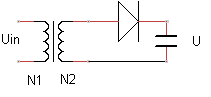Uin = 200 Veff
C = 4700 µF
N1 = 1000 wdgs
N2 = 100 wdgs

What is the value of U (onloaded)?

A. ± 2,800 V   B. ± 2,000 V   C. ± 20 V   D. ± 28 V

A. f = T/1   B. f = 2ΠR   C. f = 1/T

A. 0 V   B. 1 V   C. 2 V   D. 3 V

4.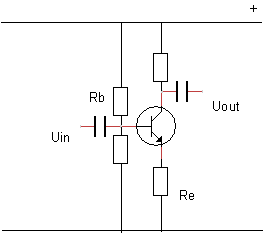This is an amplifier with:

A. High voltage amplification

B. Voltage amplification with value = 0

C. Low voltage amplification

5.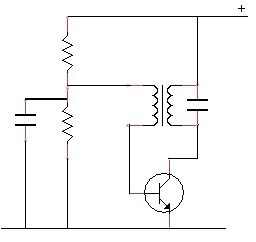This configuration is a:

A. mixing circuit

B. high frequency amplifier

C. oscillator

D. modulator

6.Which transistor conducts?

A.
B.
C.
D.

7.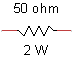What is the maximum allowable current?
A. 25 mA
B. 40 mA
C. 200 mA

8.Current I is:

A. 0 Amp
B. 1 Amp
C. 2 Amp
D. 4 Amp

9.Which symbol represents a current source:

A, B, C or D

10.The screen grid voltage -Ug is calculated with the following formula:

A. Ig * RL .
B. Ua / Ra .
C. Ua / (Ra+Rk) .
D. Ia * Rk .

A. 34 kHz   B. 25 kHz   C. 16 kHz   D. 8 kHz.

A. rapid variation of signals   B. square waves   C. slow variation of signals.

A. binaire code   B. analogue code   C. hexadecimal code   D. 6 tone code.

A. AM   B. CW   C. BPSK   D. FSK

15. Ohm's law. Some elementary questions. Chapter 1. in the F book from the VERON.

What is correct?

a. The voltage is propotional to the current and the resistance.

b. The resistance is proportional to the voltage and inversely proportional to the current

c. The resistance is inversely proportional to the voltage and proportional to the current

d. The resistance is inversely proportional to the current and the voltage.

16.

a. 10 Amp.

b. 2 Amp.

c. 1.5 Amp.

d. 0 Amp.

17.

a. 8 Ω

b. 4 Ω

c. 2 Ω

d. 0.5 Ω

18.

The 'unit' for energy is?

a. Volt; b. watt; c. watt/sec.; d. Joule

19.

a. 0.004 Watt; b. 0.04 Watt, c. 0.4 Watt; d. 4 Watt

20.

a. ½ x

b. 2 x

c. 4 x

d. remains the same.

21.

U1 = 4 V.; U2 = 8 V. In resistor R is 4 Watt being dissipated.
When S is switched, how much Watt is than dissipated in R ?

a. 1 W
b
. 2 W
c.
8 W
d
. 16 W

22.

a. 10 V

b. 9 V

c. 1 V

d. 0 V

23
.

What is the short-circuit current in the above picture ?

a. infinite   b. 10 A    c. 9 A    d. 1 A

24.

a. 5 A

b. 3.5 A

c. 2 A

d. 1 A

25
.

What is the unit of electric charge ?

a. Φ    b. Ampère   c. Coulomb    d. Joule

26.

A battery of 40 Ah is being discharged in 10 hours. The average current was:

a. 400 A   b. 40 A   c. 4 A   d. 0.4 A

27.

Why is a very high voltage being used when transporting electricity over long distances?

a. Then the current is small

b. the loss of energy is small

c. this is just the way it is being done

28.

How often does one need to use one's call sign during a QSO ?

a. at the beginning and at the end of a QSO

b. every time when the microphone (or key) is passed on to the other party

c. every five minutes

d. this is just for dumbo's

29.

a. 0 V.

b. 2 V.

c. 4 V.

d. 8 V.

30.

a. 0.5 A

b. 1 A

c. 1.5 A

d. 2 A

31.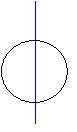The internal resistance of this power source is:

a. very high
b. dependant of the current
c. low
d. zero

32.

The characters R F are spelled with the NATO alphabet as:

a. Radio - Fox ; b. Romeo - Fox ; c. Radio - Foxtrot ; d. Romeo - Foxtrot

33.

A radio-electrical transmission installation consists of:

a. A transmitter with its antenna ; b. the transmitter ; c. the transceiver ; d. the transceiver with its antenna.

a. V/m    b. A/m    c. V.m.   d. Am

35.

a. V/m    b. Watt/m    c. Watt/m2    d. A.V/m

36.

a. 8 Volt
b. 5,6 Volt
c. 4 Volt
d. 2 Volt

37.How much power is used in this diagram with an open and a closed switch?

a. 4 and 2 watt
b. 4 and 4 watt
c. 4 and 16 watt
d. 4 and 8 watt

38.E = 20 Volt; I = 1 A. URi = 2 Volt; URu = 18 Volt. What is the value of Ri ?
a. 0.5 Ω
b. 1 Ω
c. 2 Ω
d. 4 Ω

39.

a. 3 km/h    b. 300,000 km/h    c. 300,000 km/s    d. 300,000,000 km/s

40.

a. the wave length    b. the delay factor    c. light    d. the conductor

a. 0 Volt

b. 7 Volt

c. 5 Volt

d. 4 Volt

a. 6 Volt   b. 4.5 Volt   c. 3 Volt    d. - 1.5 Volt

b. 100 Hz

c. 500 Hz

d. 1 kHz

44.UAB = ?

a.
15 Volt
b. 10 Volt
c. 6 Volt
d. 16 Volt

45.
The effective voltage of a sinus AC with an amplitude of 20 Volts is (approx.) ?
a. 6.4 Volt    b. 7 Volt    c. 12.8 Volt    d. 14 Volt

46.
Is a ham operator allowed to work with other CB operators on 27 MHz ?
a. as compliant with the regulations: only with other ham operators.
b. only if his transmission power stays within the power limits
c. of course
d. only if approved equipment (CE) for CB usage is being used.

a. 100 Watt FM transmitter with 5 kHz frequency sweep
b. 50 Watt SSB transmitter
c. 25 Watt SSB transmitter
d. 400 Watt SSB transmitter

a. 15    b. 12.5    c. 2    d. 0.5

a. 15 kHz    b. 7.5 kHz    c. 5 kHz    d. 2.5 kHz

a. FSK    b. AM    c. CW    d. PM

51.
Herea signal gets digitized. What is the right order?

a. 1. sampler   2. D/A converter   3. reconstruction filter
b. 1. anti aliassing filter   2. sampler   3. A/D converter
c. 1. anti aliassing filter   2. sampler   4. D/A converter
d. 1. sampler   2. anti aliassing filter   3. A/D converter

a. it has a number sequence from 1 to 10
b. it has 6 numbers and 6 characters
c. it is a logarithmic system
d. it represents two switching levels

a. an analogue method    b. switching using BPSK    c. switching using FSK    d. switching using PSK on different levels

a. 128 positions    b. 64 positions    c. 32 positions    d. 16 positions

a. kHz    b. bits/second    c. byte/second    d. bit * second

56.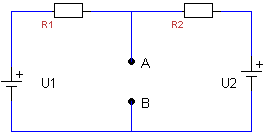U1 = 5V
U2 = 5V

R1 = 4Ω
R2 = 6Ω

UAB = ?

a. 0 Volt    b. 2 Volt    c. 4 Volt    d. 18 Volt

a. 10 Volt

b. 8 Volt

c. 2 Volt

d. 0 Volt

58.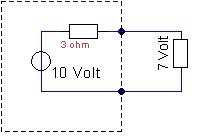What is the value of the short circuit current?

a. 0 A.
b. 1 A.
c. 3.33 A.
d. 7.4 A.

a. there is no error correction   b. each character is sent twice   c. the other 'party' gives an acknowledgement    d. is only used with FSK

a. is the PEP 400 Watts   b. is the PEP 100 Watt   c. is the PEP 50 Watt   d. is the PEP 25 Watt

a. quantisising noise   b. brown noise   c. cosmic noise   d. white noise.

a. to obtain the different voltage levels required by the A/D converter  b. to allow the phase jumps in the HF signal   c. to avoid quantisising noise    d. with no sampling one gets aliassing.

63.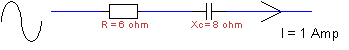How much power is dissipated in this configuration ?

a. 6 Watt    b. 8 Watt    c. 10 Watt    d. 14 Watt

64.Which statement is correct?
a. the current and  voltage are in phase
b. the voltage is leading the current
c. the current is leading the voltage
d. the current lags the voltage

a. C = 1/2∏f.Xc    b. C = 2∏fc    c.  C = 2∏F/Xc    d. C = Xc/2∏f

a. 0 V.    b. 2 V.    c. 4 V.    d. 6 V.

67
.C1 = C2 = 10µF. What is the replacement value Crv ?

a. 20µF    b. 10µF    c. 5 µF    d. 2.5 µF

68.Crv = ?  (RV stands for replacement value)

a. 2µF
b. 0.8 µF
c. 8µF
d. 5µF

a) equal to R   b) XL2 + R2   c) 1/XL2 + R2   d) very high

a) XL = 1/2∏f.L   b) XL = 2∏f/L   c) XL = 2∏f.L
d)
XL = ω/2∏f.L

a) aluminium    b) wood    c) tinplate    d) magnetic tinplate

a) Henry    b) A/m    c) Am    d) V/m

91.

a. give priority to all other users
b. give priority to all other secundary users
c. give priority to primary users
d. doesn't matter whata. Q = c.u
b. c = u/Q
c. c= Q/u2
d. c = Q/u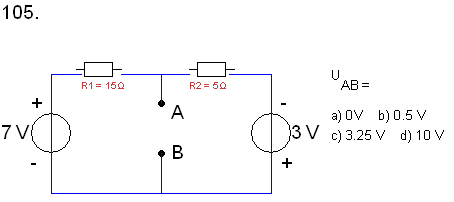a) 0 W   b) 3 mW    c) 10 mW    d) 13 mW

a) 0 W    b) 4 W    c) 5000 W    d) 5004 W.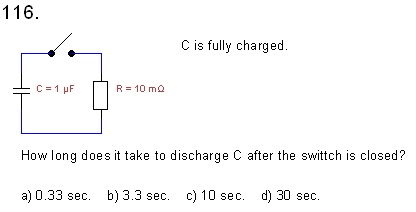a) at a capacitor the current leads the voltage
b) at a capacitor the voltage leads the current
c) at a coil the current leads the voltage
d) none of the above is correct.

a) 1 cell   b) 6 cells    c) 10 cells    d) 12 cells

122.

a) 10 Hz    b) 50 Hz    c) 1 kHz    d) 15 kHz

a) allowed    b) allowed unless it is a commercial message    c) not allowed

126.

a) allowed    b) not allowed    c) allowed, provided it complies to certain specifications    d) allowed, provided it is approved by the appropriate government agency

a) Collpits    b) Hartley    c) crystal    d) VCO

Note: Voltage reduced i.e. less positive (+); Voltage increases i.e. more positive (+).

a) 3 W    b) 180 W   c) 675 W    d) 2700 W

a) 0.5    b) 2    c) 12.5    d) 15

a) not change    b) increase four (4) times    c) increase two (2) times
d) increase ten (10) times

a) The power amplifier (PA) of the transmitter is set in class C.
b) The squelch of the receiver is set to tight
c) the transmitter has a sweep that is too large
d) the transmitter has a sweep that is too small

a) the tuning at the input    b) the balance mixer
c) a high quality crystal filter in the MF circuit   d)  a DSP audio unit.

a) a diode detector    b) FM-discriminator    c) a product-detector
d) a flank-detector

a) putting a low pass filter in the modulator
b) putting a low pass filter in the antenna of the transmitter
c) putting a high pass filter behind the transmitter
d) to only work FM

a) install a pre-amplifier near the antenna
b) install a pre-amplifier near the receiver
c) apply a large MF amplification
d) apply a large LF amplification

a) thermic noise    b) cosmic noise    c) white noise    d) brown noise

Here I end my contribution to get familiar with the F license stuff, knowing there are many more items I could (or should) have addressed.